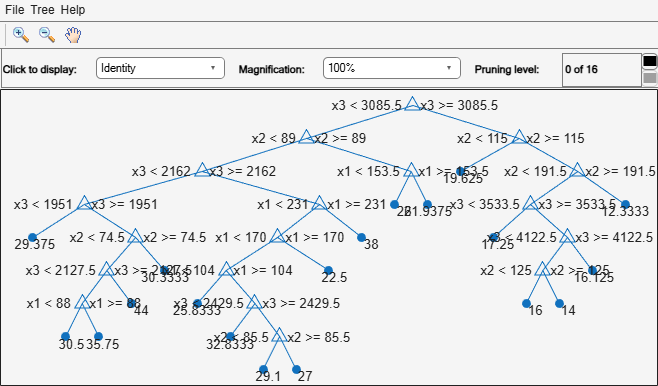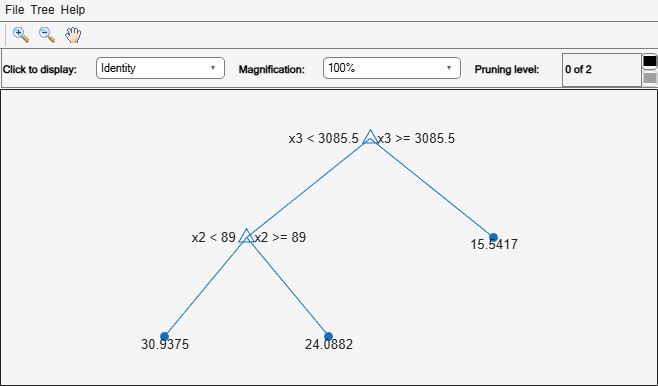# cvloss

Class: RegressionTree

Regression error by cross validation

## Syntax

```E = cvloss(tree) [E,SE] = cvloss(tree) [E,SE,Nleaf] = cvloss(tree) [E,SE,Nleaf,BestLevel] = cvloss(tree) [E,...] = cvloss(tree,Name,Value) ```

## Description

`E = cvloss(tree)` returns the cross-validated regression error (loss) for `tree`, a regression tree.

```[E,SE] = cvloss(tree)``` returns the standard error of `E`.

```[E,SE,Nleaf] = cvloss(tree)``` returns the number of leaves (terminal nodes) in `tree`.

```[E,SE,Nleaf,BestLevel] = cvloss(tree)``` returns the optimal pruning level for `tree`.

`[E,...] = cvloss(tree,Name,Value)` cross validates with additional options specified by one or more `Name,Value` pair arguments. You can specify several name-value pair arguments in any order as `Name1,Value1,…,NameN,ValueN`.

## Input Arguments

expand all

Trained regression tree, specified as a `RegressionTree` object constructed using `fitrtree`.

### Name-Value Pair Arguments

Specify optional comma-separated pairs of `Name,Value` arguments. `Name` is the argument name and `Value` is the corresponding value. `Name` must appear inside quotes. You can specify several name and value pair arguments in any order as `Name1,Value1,...,NameN,ValueN`.

Pruning level, specified as the comma-separated pair consisting of `'Subtrees'` and a vector of nonnegative integers in ascending order or `'all'`.

If you specify a vector, then all elements must be at least `0` and at most `max(tree.PruneList)`. `0` indicates the full, unpruned tree and `max(tree.PruneList)` indicates the completely pruned tree (i.e., just the root node).

If you specify `'all'`, then `cvloss` operates on all subtrees (i.e., the entire pruning sequence). This specification is equivalent to using `0:max(tree.PruneList)`.

`cvloss` prunes `tree` to each level indicated in `Subtrees`, and then estimates the corresponding output arguments. The size of `Subtrees` determines the size of some output arguments.

To invoke `Subtrees`, the properties `PruneList` and `PruneAlpha` of `tree` must be nonempty. In other words, grow `tree` by setting `'Prune','on'`, or by pruning `tree` using `prune`.

Example: `'Subtrees','all'`

Data Types: `single` | `double` | `char` | `string`

Tree size, specified as the comma-separated pair consisting of `'TreeSize'` and one of the following:

• `'se'``cvloss` uses the smallest tree whose cost is within one standard error of the minimum cost.

• `'min'``cvloss` uses the minimal cost tree.

Number of folds to use in a cross-validated tree, specified as the comma-separated pair consisting of `'KFold'` and a positive integer value greater than 1.

Example: `'KFold',8`

## Output Arguments

expand all

Cross-validation mean squared error (loss), returned as a vector or scalar depending on the setting of the `Subtrees` name-value pair.

Standard error of `E`, returned as vector or scalar depending on the setting of the `Subtrees` name-value pair.

Number of leaf nodes in `tree`, returned as a vector or scalar depending on the setting of the `Subtrees` name-value pair. Leaf nodes are terminal nodes, which give responses, not splits.

Best pruning level as defined in the `TreeSize` name-value pair, returned as a scalar whose value depends on `TreeSize`:

• If `TreeSize` is `'se'`, then `BestLevel` is the largest pruning level that achieves a value of `E` within `SE` of the minimum error.

• If `TreeSize` is `'min'`, then `BestLevel` is the smallest value in `Subtrees`.

## Examples

expand all

Compute the cross-validation error for a default regression tree.

Load the `carsmall` data set. Consider `Displacement`, `Horsepower`, and `Weight` as predictors of the response `MPG`.

```load carsmall X = [Displacement Horsepower Weight];```

Grow a regression tree using the entire data set.

`Mdl = fitrtree(X,MPG);`

Compute the cross-validation error.

```rng(1); % For reproducibility E = cvloss(Mdl)```
```E = 27.6976 ```

`E` is the 10-fold weighted, average MSE (weighted by number of test observations in the folds).

Apply k-fold cross validation to find the best level to prune a regression tree for all of its subtrees.

Load the `carsmall` data set. Consider `Displacement`, `Horsepower`, and `Weight` as predictors of the response `MPG`.

```load carsmall X = [Displacement Horsepower Weight];```

Grow a regression tree using the entire data set. View the resulting tree.

```Mdl = fitrtree(X,MPG); view(Mdl,'Mode','graph')```Compute the 5-fold cross-validation error for each subtree except for the first two lowest and highest pruning level. Specify to return the best pruning level over all subtrees.

```rng(1); % For reproducibility m = max(Mdl.PruneList) - 1```
```m = 15 ```
`[~,~,~,bestLevel] = cvloss(Mdl,'SubTrees',2:m,'KFold',5)`
```bestLevel = 14 ```

Of the `15` pruning levels, the best pruning level is `14`.

Prune the tree to the best level. View the resulting tree.

```MdlPrune = prune(Mdl,'Level',bestLevel); view(MdlPrune,'Mode','graph')```## Alternatives

You can construct a cross-validated tree model with `crossval`, and call `kfoldLoss` instead of `cvloss`. If you are going to examine the cross-validated tree more than once, then the alternative can save time.

However, unlike `cvloss`, `kfoldLoss` does not return `SE`, `Nleaf`, or `BestLevel`.